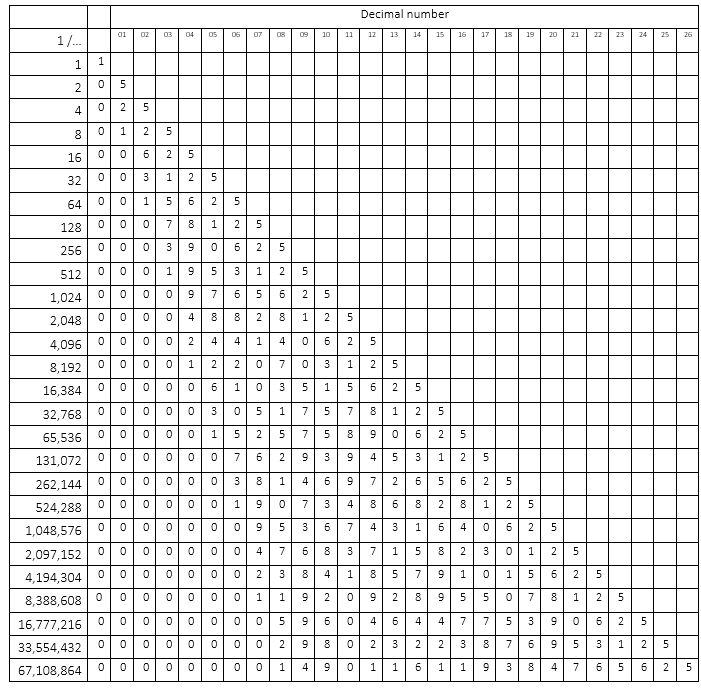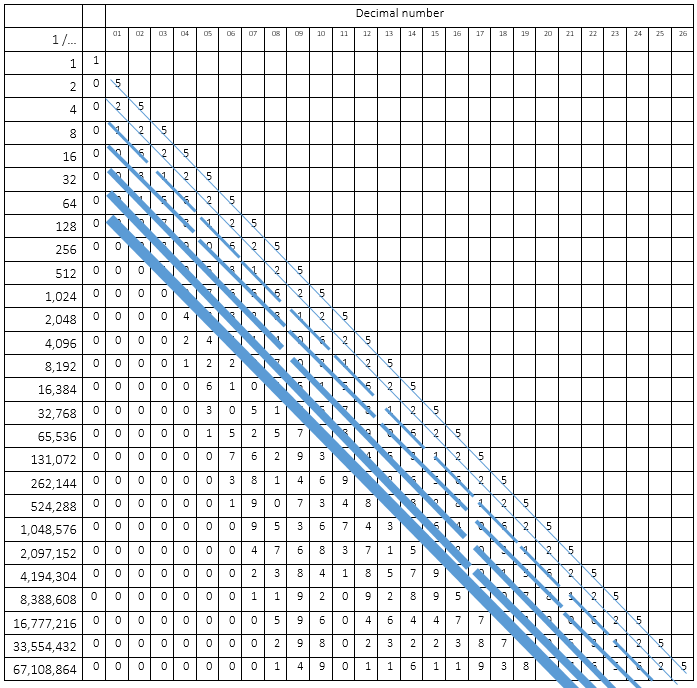# The tree of dividing 1 by the powers of 2

I wondered what (or if a) pattern emerges when I divided 1 by 2, that answer by 2 again, that answer by 2, and so on (so basically 1/ by the powers of 2). Would I find some set of numbers or some “random” sequence of numbers? Many calculators weren’t able to show the numbers that far after the decimal point, so at the 26th decimal I stopped the calculations (+ it costed some (design) effort). Nevertheless, I would loved to go through the 32nd decimal, I will show later why.You can quickly spot the first pattern, every number ends at a 5, then a 25, and then either a 125, or a 625. And this continued on and on, resulting in a more complex pattern. I decided to make some lines across the table, which you can find in the next figure to show the pattern.What to learn?
When drawing it like this, the pattern seems like the fractal Cantor-set, or the binary tree, but a little bit different. There are different things you can learn from this pattern that emerges.

The power of patterns
You can see from the pattern that you start with a pattern length from 1 (the 5’s), 1 (the 2’s), 2 (1,6), 4 (0,3,5,8), 8 (0,1,7,9,5,6,2,4), and so on. I came to the 16’s decimals which is the end of a pattern of decimals. I am sure that the 32nd decimal will also be the end of a pattern. So in fact, the decimal patterns are the same length as each power of two.

Numbers become predictable
I would assume that the longer the numbers become, the less “predictable” each number becomes. But in fact, each number is part of a larger set/structure. However, the set of numbers within a pattern (like 0,3,5,8) don’t look that obviously connected to each other. Nevertheless, it is quite weird to realize that the further the numbers behind the decimal, the more they become “predictable” using the pattern. You know each number will end at 25, every 1 divided by 8, 32, 128, and so on at “125” (the second in place, expect the 2nd itself), every 1 divided by 32, 512,  8192 at “3125” (each fourth in place, expect the fourth itself), and so on. You can make even more pretty patterns using this sequences. But what I want to say, is that you can know the last decimals of each numbers quite easily, very, very far behind zero. And the further you go, the more predictable each number becomes.